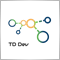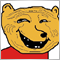5244

I have converted an mt4 indicator to mt5 . The code and the logic is exactly the same but the out come is completely wrong on MT5. I am attaching the MT5 code and two pictures one from MT4 which is the correct outcome of the indicator and a picture with the wrong outcome from MT5. Could someone please help out
Files:5244

#property strict
#property version "1.0"
#property icon      "WeisPipWave60x60.ico"
#property indicator_separate_window
#property indicator_minimum 0
#property indicator_buffers 5
#property indicator_plots   2
//--- plot upVolume
#property indicator_label1  "upPips"
#property indicator_type1   DRAW_HISTOGRAM
#property indicator_color1  clrGreen
#property indicator_style1  STYLE_SOLID
#property indicator_width1  2
//--- plot dnVolume
#property indicator_label2  "dnPips"
#property indicator_type2   DRAW_HISTOGRAM
#property indicator_color2  clrFireBrick
#property indicator_style2  STYLE_SOLID
#property indicator_width2  2

//--- input parameters
input int      Difference = 120;

input string Alert_On_Volume = "Alert on Volume>VolSize on TriggerCandle";
input int    TriggerCandle      = 1;
input double PipWaveSize=100;
input bool   EnableNativeAlerts = false;
input bool   EnableSoundAlerts  = false;
input bool   EnableEmailAlerts  = false;
input string SoundFileName      = "alert.wav";

datetime LastAlertTimeP = D'01.01.1970';
int LastAlertDirectionP = 0;

//--- indicator buffers

double         barDirection[];
double         trendDirection[];
double         waveDirection[];
double         upPipBuffer[];
double         dnPipBuffer[];

double         pipTracker=0;

double         highestHigh = EMPTY_VALUE;
double         lowestLow   = EMPTY_VALUE;
int            hhBar = EMPTY_VALUE;
int            llBar = EMPTY_VALUE;

//+------------------------------------------------------------------+
//| Custom indicator initialization function                         |
//+------------------------------------------------------------------+
int OnInit()
{
//--- indicator buffers mapping

SetIndexBuffer(0, upPipBuffer);
SetIndexBuffer(1, dnPipBuffer);
SetIndexBuffer(2, trendDirection);
SetIndexBuffer(3, waveDirection);
SetIndexBuffer(4, barDirection);
//  ArraySetAsSeries(upPipBuffer,true);
//  ArraySetAsSeries(dnPipBuffer,true);
//ArraySetAsSeries(trendDirection,true);
///ArraySetAsSeries(waveDirection,true);
//ArraySetAsSeries(barDirection,true);
//  SetLevelValue(1,PipWaveSize);
//  SetLevelStyle(STYLE_DASH,1);
//---
return(INIT_SUCCEEDED);
}
//+------------------------------------------------------------------+
//| Custom indicator deinitialization function                       |
//+------------------------------------------------------------------+

//+------------------------------------------------------------------+
//| Custom indicator iteration function                              |
//+------------------------------------------------------------------+
int OnCalculate(const int rates_total,
const int prev_calculated,
const datetime &time[],
const double &open[],
const double &high[],
const double &low[],
const double &close[],
const long &tick_volume[],
const long &volume[],

{
//---
// Only compute bars on new bar
if (rates_total == prev_calculated) return(rates_total);

int limit = rates_total-1 ;
Print("ratestotal ", rates_total, " prev_r ", prev_calculated);
int  waveChangeBar = limit-1;

// Initialise values
if (highestHigh == EMPTY_VALUE) highestHigh =close[waveChangeBar];
if (lowestLow ==EMPTY_VALUE) lowestLow = close[waveChangeBar];
if (hhBar == EMPTY_VALUE) hhBar = waveChangeBar;
if (llBar ==EMPTY_VALUE) llBar = waveChangeBar;

for(int i=limit-1; i>=0; i--) {

// Determine this bar's direction
if (close[i] - close[i+1] >  0) barDirection[i] =  +1;    // current close higher
if (close[i] - close[i+1] == 0) barDirection[i] =  0;    // current close equal
if (close[i] - close[i+1] <  0)  barDirection[i]= -1;    // current close lower
Print(barDirection[i]);
if (barDirection[limit]   == EMPTY_VALUE) barDirection[limit]   = barDirection[i];
if (trendDirection[limit] == EMPTY_VALUE) trendDirection[limit] = barDirection[i];
if (waveDirection[limit]  == EMPTY_VALUE) waveDirection[limit]  = barDirection[i];

// Determine highset high and lowest low
if (close[i] > highestHigh) {
highestHigh = close[i];
hhBar = i;
}
else if (close[i] < lowestLow) {
lowestLow = close[i];
llBar = i;
}
// Determine if this bar has started a new trend
if ((barDirection[i] != 0) && (barDirection[i] != barDirection[i+1]))
trendDirection[i] = barDirection[i];
else  trendDirection[i] = trendDirection[i+1];

// Determine if this bar has started a new wave
double waveTest =0.0;
if (waveDirection[i+1] == +1  ) {
waveTest = highestHigh ;
}
if (waveDirection[i+1] == -1  ) {
waveTest = lowestLow;
}
double waveDifference = (MathAbs(waveTest - close[i])) * MathPow(10, _Digits);;
//  Print(waveDifference);
if (trendDirection[i] != waveDirection[i+1]) {
if (waveDifference >= Difference ) waveDirection[i] = trendDirection[i];
else waveDirection[i] = waveDirection[i+1];
}
else waveDirection[i] = waveDirection[i+1];

// Determine if we have started a new wave
if (waveDirection[i] != waveDirection[i+1] ) {    //&& close[i] !=close[i+1]
if (waveDirection[i] == 1) {
highestHigh = close[i];
hhBar = i;
waveChangeBar = llBar;
}
else {
lowestLow = close[i];
llBar = i;
waveChangeBar = hhBar;
}

Print(i, " limit ", limit, "highest high " ,highestHigh, " hhbar " , hhBar, " trend ", trendDirection[i], " wave ",waveDirection[i], " bardir ",barDirection[i]);
pipTracker=0;
for (int k=waveChangeBar-1; k>=i; k--)     {

pipTracker +=(open[k]-close[k])*MathPow(10, _Digits)/10;
if (waveDirection[i] == +1) {
dnPipBuffer[k]=0;
upPipBuffer[k]=MathAbs(pipTracker);

}
if (waveDirection[i] == -1) {

upPipBuffer[k]=0;
dnPipBuffer[k]=MathAbs(pipTracker);
}
}

}
else {
pipTracker+=(open[i]-close[i])*MathPow(10, _Digits)/10;
}

// Set the indicators
if (waveDirection[i] ==  +1) {
dnPipBuffer[i]=0;
upPipBuffer[i]=MathAbs(pipTracker);

}
if (waveDirection[i] == -1) {

upPipBuffer[i]=0;
dnPipBuffer[i]=MathAbs(pipTracker);

}
Print(dnPipBuffer[i]);

}
//if (((TriggerCandle > 0) && (Time > LastAlertTimeP)) || (TriggerCandle == 0))
//{
//string Text;

//if ((upPipBuffer[TriggerCandle] > PipWaveSize) && (upPipBuffer[TriggerCandle+1] < PipWaveSize) && ((TriggerCandle > 0) || ((TriggerCandle == 0) && (LastAlertDirectionP != 1))))

//{
//      Text = _Symbol+" "+ IntegerToString(Period())+" HIGH UP PIP WAVE " + " - "+TimeToString(Time);
//      if (EnableEmailAlerts) SendMail(_Symbol+ " HIGH UP PIP WAVE ", Text);
//      if (EnableSoundAlerts) PlaySound(SoundFileName);
//      LastAlertTimeP = Time;
//      LastAlertDirectionP = 1;
//}
// Down Arrow Alert
//if ((dnPipBuffer[TriggerCandle] > PipWaveSize)&& (dnPipBuffer[TriggerCandle+1] < PipWaveSize) && ((TriggerCandle > 0) || ((TriggerCandle == 0) && (LastAlertDirectionP != -1))))
//{
//      Text =_Symbol+ " "+ IntegerToString(Period())+" HIGH DN PIP WAVE  " + " - "+TimeToString(Time);
//      if (EnableEmailAlerts) SendMail(_Symbol+ " HIGH DN PIP WAVE ", Text);
//      if (EnableSoundAlerts) PlaySound(SoundFileName);
//      LastAlertTimeP = Time;
//      LastAlertDirectionP = -1;
//}
//}

//--- return value of prev_calculated for next call
return(rates_total);
}1081

Rates index goes the other way in MQL5.5244

kypa:
Rates index goes the other way in MQL5.
Thanks for your reply. Could you please be more specific, do I need to loop differently?1081

In MQL4 the last bar is Bar, in MQL5 it is Bar[rates_total-1]. So if you want to start from most left loop should be 0 to rates_total. Also you probably don't need to recalculate the whole thing every time. Additionally if new history is added to chart prev_calculated is set to 0, you should probably handle that too.5244

kypa:
In MQL4 the last bar is Bar, in MQL5 it is Bar[rates_total-1]. So if you want to start from most left loop should be 0 to rates_total. Also you probably don't need to recalculate the whole thing every time. Additionally if new history is added to chart prev_calculated is set to 0, you should probably handle that too.
Got it. Thank you.